Home | | Physics 11th std | Collisions

# Collisions

Collision is a common phenomenon that happens around us every now and then.

COLLISIONS

Collision is a common phenomenon that happens around us every now and then. For example, carom, billiards, marbles, etc.,. Collisions can happen between two bodies with or without physical contacts.

Linear momentum is conserved in all collision processes. When two bodies collide, the mutual impulsive forces acting between them during the collision time (∆t) produces a change in their respective momenta. That is, the first body exerts a force12 on the second body. From Newton’s third law, the second body exerts a force21 on the first body. This causes a change in momentum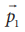andof the first body and second body respectively. Now, the relations could be written as,Adding equation (4.44) and equation (4.45), we get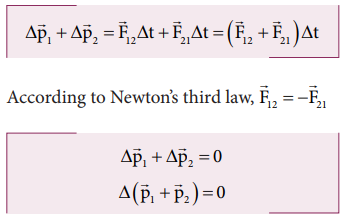Dividing both sides by t and taking limit ∆t →0 , we getThe above expression implies that the total linear momentum is a conserved quantity. Note: The momentum is a vector quantity. Hence, vector addition has to be followed to find the total momentum of the individual bodies in collision.

## Types of Collisions

In any collision process, the total linear momentum and total energy are always conserved whereas the total kinetic energy need not be conserved always. Some part of the initial kinetic energy is transformed to other forms of energy. This is because, the impact of collisions and deformation occurring due to collisions may in general, produce heat, sound, light etc. By taking these effects into account, we classify the types of collisions as follows:

a. Elastic collision

b. Inelastic collision

### a. Elastic collision

In a collision, the total initial kinetic energy of the bodies (before collision) is equal to the total final kinetic energy of the bodies (after collision) then, it is called as elastic collision. i.e.,

Total kinetic energy before collision = Total kinetic energy after collision

### b. Inelastic collision

In a collision, the total initial kinetic energy of the bodies (before collision) is not equal to the total final kinetic energy of the bodies (after collision) then, it is called as inelastic collision. i.e.

Total kinetic energy before collision ≠ Total kinetic energy after collisionEven though kinetic energy is not conserved but the total energy is conserved. This is because the total energy contains the kinetic energy term and also a term Q , which includes all the losses that take place during collision. Note that loss in kinetic energy during collision is transformed to another form of energy like sound, thermal, etc. Further, if the two colliding bodies stick together after collision such collisions are known as completely inelastic collision or perfectly inelastic collision. Such a collision is found very often. For example when a clay putty is thrown on a moving vehicle, the clay putty (or Bubblegum) sticks to the moving vehicle and they move together with the same velocity.## Elastic collisions in one dimension

Consider two elastic bodies of masses m1 and m2 moving in a straight line (along positive x direction) on a frictionless horizontal surface as shown in figure 4.16.In order to have collision, we assume that the mass m1 moves faster than mass m2 i.e., u1 > u2. For elastic collision, the total linear momentum and kinetic energies of the two bodies before and after collision must remain the same.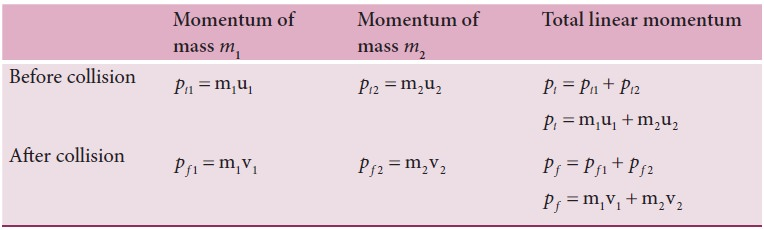From the law of conservation of linear momentum,

Total momentum before collision ( pi) = Total momentum after collision ( pf )Further,For elastic collision,

Total kinetic energy before collision KEi = Total kinetic energy after collision KE fAfter simplifying and rearranging the terms,Using the formula a2 - b2 = (a+b)( a-b) , we can rewrite the above equation asDividing equation (4.49) by (4.47) gives,This means that for any elastic head on collision, the relative speed of the two elastic bodies after the collision has the same magnitude as before collision but in opposite direction. Further note that this result is independent of mass.

Rewriting the above equation for  v1 and v2,### To find the final velocities v1 and v2:

Substituting equation (4.52) in equation (4.47) gives the velocity of m1 asSimilarly, by substituting (4.51) in equation (4.47) or substituting equation (4.53) in equation (4.52), we get the final velocity of m2 asCase 1: When bodies has the same mass i.e., m1 = m2,The equations (4.55) and (4.56) show that in one dimensional elastic collision, when two bodies of equal mass collide after the collision their velocities are exchanged.

Case 2:  When bodies have the same mass i.e., m1 = m2 and second body (usually called target) is at rest (u2 = 0), By substituting m1 = m2 and u2 = 0 in equations  4.53) and equations (4.54) we get,Equations (4.57) and (4.58) show that when the first body comes to rest the second body moves with the initial velocity of the first body.

Case 3:

The first body is very much lighter than the second bodythen the ratio m1/m2 ≈ 0 and also if the target is at rest ( u2=0)

Dividing numerator and denominator of equation (4.53) by m2, we getSimilarly,

Dividing numerator and denominator of equation (4.54) by m2, we getThe equation (4.59) implies that the first body which is lighter returns back (rebounds) in the opposite direction with the same initial velocity as it has a negative sign. The equation (4.60) implies that the second body which is heavier in mass continues to remain at rest even after collision. For example, if a ball is thrown at a fixed wall, the ball will bounce back from the wall with the same velocity with which it was thrown but in opposite direction.

Case 4:

The second body is very much lighter than the first bodythen the ratio m2/m1 ≈ 0 and also if the target is at rest ( u2=0)

Dividing numerator and denominator of equation (4.53) by m1, we getSimilarly,

Dividing numerator and denominator of equation (4.58) by m1, we getThe equation (4.61) implies that the first body which is heavier continues to move with the same initial velocity. The equation (4.62) suggests that the second body which is lighter will move with twice the initial velocity of the first body. It means that the lighter body is thrown away from the point of collision.

## Perfect inelastic collision

In a perfectly inelastic or completely inelastic collision, the objects stick together permanently aft er collision such that they move with common velocity. Let the two bodies with masses m1 and m2  move with initial velocities u1 and u2 respectively before  collision. Aft er perfect inelastic collision both the objects move together with a common velocity v as shown in Figure (4.17).

Since, the linear momentum is conserved during collisions,

m1u1 + m2u2 = (m1 + m2)vThe common velocity can be computed by## Loss of kinetic energy in perfect inelastic collision

In perfectly inelastic collision, the loss in kinetic energy during collision is transformed to another form of energy like sound, thermal, heat, light etc. Let KEi be the total kinetic energy before collision and KEf be the total kinetic energy after collision.

Total kinetic energy before collision,Total kinetic energy after collision,Then the loss of kinetic energy is Loss of KE, Q = KE i KEfSubstituting equation (4.63) in equation (4.66), and on simplifying (expand v by using the algebra (a+b) 2 = a2+b2+2ab, we get## Coefficient of restitution (e)

Suppose we drop a rubber ball and a plastic ball on the same floor. The rubber ball will bounce back higher than the plastic ball. This is because the loss of kinetic energy for an elastic ball is much lesser than the loss of kinetic energy for a plastic ball. The amount of kinetic energy after the collision of two bodies, in general, can be measured through a dimensionless number called the coefficient of restitution (COR).

It is defined as the ratio of velocity of separation (relative velocity) after collision to the velocity of approach (relative velocity) before collision, i.e.,In an elastic collision, we have obtained the velocity of separation is equal to the velocity of approach i.e.,This implies that, coefficient of restitution for an elastic collision, e =1. Physically, it means that there is no loss of kinetic energy after the collision. So, the body bounces back with the same kinetic energy which is usually called as perfect elastic.

In any real collision problems, there will be some losses in kinetic energy due to collision, which means e is not always equal to unity. If the ball is perfectly plastic, it will never bounce back and therefore their separation of velocity is zero after the collision. Hence, the value of coefficient of restitution, e = 0.

In general, the coefficient of restitution for a material lies between 0 < e <1.

Solved Example Problems for collision

## Solved Example Problems for Elastic collisions in one dimension

### Example 4.20

A lighter particle moving with a speed of 10 m s-1 collides with an object of double its mass moving in the same direction with half its speed. Assume that the collision is a one dimensional elastic collision. What will be the speed of both particles after the collision?

### SolutionLet the mass of the fi rst body be m which  moves with an initial velocity, u1 = 10 m s-1.

Therefore, the mass of second body is 2m and its initial velocity is u2 = ½ u1 = ½(10ms-1)

Then, the fi nal velocities of the bodies can be calculated from the equation (4.53) and equation (4.54)As the two speeds v1and v2 are positive, they move in the same direction with the velocities, 3.33 m s−1 and 8.33 m s−1 respectively.

## Solved Example Problems for Perfect inelastic collision

### Example 4.21

A bullet of mass 50 g is fired from below into a suspended object of mass 450 g. The object rises through a height of 1.8 m with bullet remaining inside the object. Find the speed of the bullet. Take g = 10 ms-2.

### Solution

m1 = 50 g = 0.05 kg; m2 = 450 g = 0.45kgThe speed of the bullet is u1. The second body is at rest u2 = 0 . Let the common velocity of the bullet and the object after the bullet is embedded into the object is v.The combined velocity is the initial velocity for the vertical upward motion of the combined bullet and the object. From second equation of motion,Substituting this in the above equation, the value of u1 is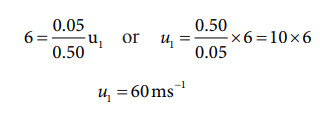## Solved Example Problems for Coefficient of restitution (e)

### Example 4.22

Show that the ratio of velocities of equal masses in an inelastic collision when one of the masses is stationary is

v1/v2 = 1-e/1+e

### Solution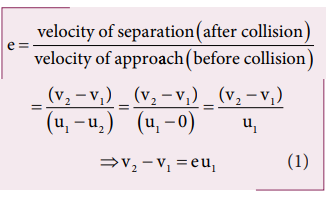From the law of conservation of linear momentum,Using the equation (2) for u1 in (1), we getStudy Material, Lecturing Notes, Assignment, Reference, Wiki description explanation, brief detail
11th Physics : UNIT 4 : Work, Energy and Power : Collisions |